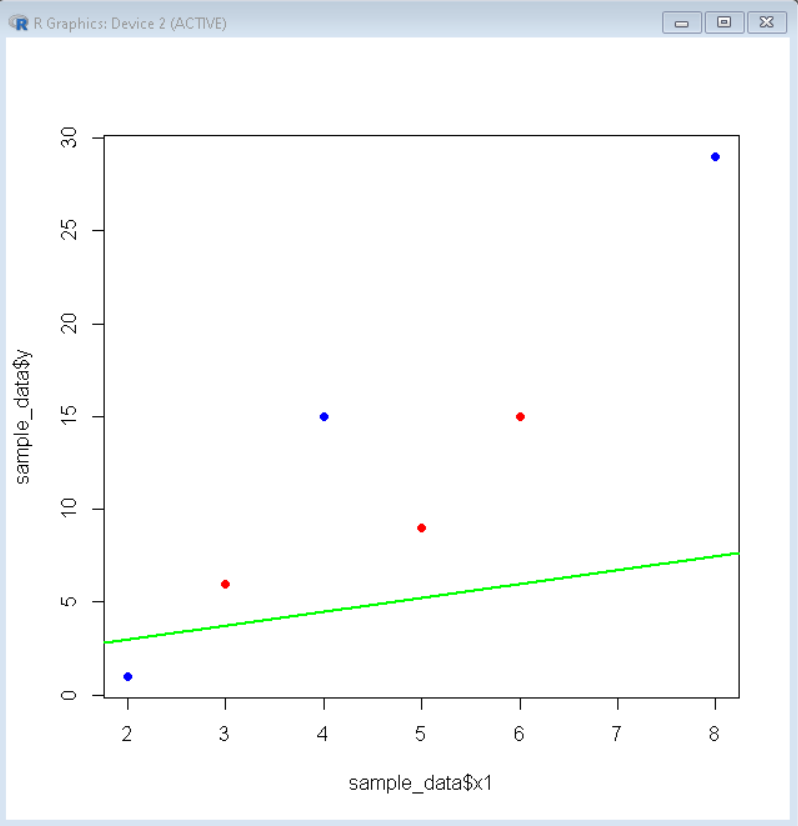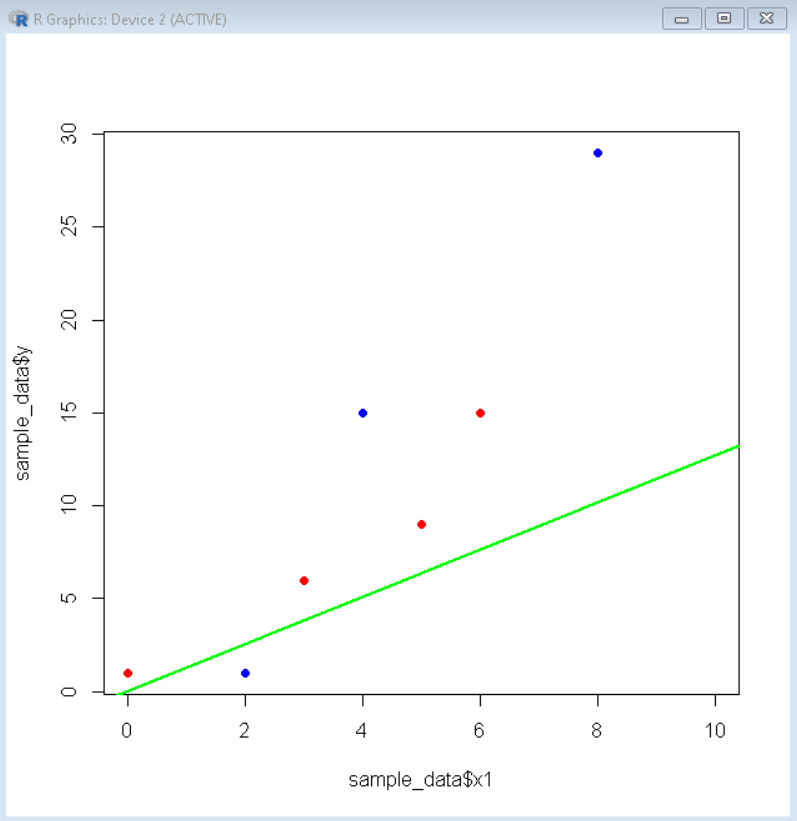Open in App
Not now

# Remove Intercept from Regression Model in R

• Last Updated : 14 Feb, 2022

In this article, we will discuss how to remove intercept from the Regression model in the R Programming Language.

## Extract intercept from the linear regression model

To extract intercept from the linear regression model in the R Language, we use the summary() function of the R Language. We first create the linear regression model using the lm() function. The lm() function is used to fit linear models to data frames in the R Language. It can be used to carry out regression, single stratum analysis of variance, and analysis of covariance to predict the value corresponding to data that is not in the data frame. Then we use the summary() function to retrieve the statistical summary of that model which also contains the information of intercept that the fitted model makes.

Syntax:

linear_model <- lm( formula, data )

summary( linear_model )

Parameter:

• formula: determines the formula for the linear model.
• data: determines the name of the data frame that contains the data.

Example: Here, is a linear regression model with intercept in the R Language.

## R

 `# sample data frame` `sample_data <- ``data.frame``( x1= ``c``(2,3,5,4,8),` `                  ``x2= ``c``(0,3,5,6,23),` `                  ``y= ``c``(1,6,9,15,29))` `  `  `# fit linear model` `linear_model <- ``lm``(y ~ x1+x2, data=sample_data)` `  `  `# view summary of linear model` `summary``(linear_model)`

Output:

```Call:
lm(formula = y ~ x1 + x2, data = sample_data)
Residuals:
1       2       3       4       5
-1.9974 -0.6673 -1.1100  4.6628 -0.8880
Coefficients:
Estimate Std. Error t value Pr(>|t|)
(Intercept)   1.5032     7.4142   0.203    0.858
x1            0.7471     2.7159   0.275    0.809
x2            0.9743     0.6934   1.405    0.295```

Here, the intercept is estimated to be 1.5032, which can be seen clearly in the coefficient section of the linear model summary.

## Visualization of Regression Model

To visualize the linear regression model in the R Language, we use the plot() function to plot the scatter plot of data points and then use the abline() pot to plot the regression line.

Syntax:

plot( datax, datay )

abline( linear_model)

Parameter:

• datax and datay: determine the value for the x-axis and y-axis variables.
• linear_model: determines the linear model for visualization.

Example: Here, is a visualization of a linear model with intercept.

## R

 `# sample data frame` `sample_data <- ``data.frame``( x1= ``c``(2,3,5,4,8),` `                  ``x2= ``c``(0,3,5,6,23),` `                  ``y= ``c``(1,6,9,15,29))` `  `  `# fit linear model` `linear_model <- ``lm``(y ~ x1+x2, data=sample_data)` `  `  `# visualize linear model` `plot``( sample_data\$x1, sample_data\$y, col= ``"blue"``, pch=16 )` `points``( sample_data\$x2, sample_data\$y, col=``"red"``, pch=16 )` `abline``(linear_model, col=``"green"``, lwd=2 )`

Output:## Remove intercept from the linear regression model

To remove the intercept from a linear model, we manually set the value of intercept zero. In this way, we may not necessarily get the best fit line but the line guaranteed passes through the origin. To set the intercept as zero we add 0 and plus sign in front of the fitting formula. This makes the intercept zero.

Syntax:

linear_model <- lm( var1 ~ 0+ formula, data )

summary( linear_model )

Parameter:

• var1: determines the variable on which data is to be fitted.
• formula: determines the formula for the linear model.
• data: determines the name of the data frame that contains the data.

Example: Here, is a linear regression model without intercept in the R Language.

## R

 `# sample data frame` `sample_data <- ``data.frame``( x1= ``c``(2,3,5,4,8),` `                  ``x2= ``c``(0,3,5,6,23),` `                  ``y= ``c``(1,6,9,15,29))` `  `  `# fit linear model` `linear_model <- ``lm``(y ~ 0+x1+x2, data=sample_data)` `  `  `# view summary of linear model` `summary``(linear_model)`

Output:

Call:

lm(formula = y ~ 0 + x1 + x2, data = sample_data)

Residuals:

1       2       3       4       5

-1.5422 -0.3795 -1.6325  4.7831 -0.8434

Coefficients:

Estimate Std. Error t value Pr(>|t|)

x1   1.2711     0.6886   1.846   0.1621

x2   0.8554     0.3056   2.799   0.0679 .

Signif. codes:  0 ‘***’ 0.001 ‘**’ 0.01 ‘*’ 0.05 ‘.’ 0.1 ‘ ’ 1

Residual standard error: 3.097 on 3 degrees of freedom

Multiple R-squared:  0.9757,    Adjusted R-squared:  0.9595

F-statistic: 60.22 on 2 and 3 DF,  p-value: 0.003789

Here, we don’t get intercept in the coefficient of summary section as the intercept is set to zero.

## Visualization of linear model without intercept

To visualize the linear model without intercept, we add zero and plus(+) sign in front of the fitting formula. Then, we use the plot() and the abline() functions to visualize the linear regression model.

Example: Here, is a plot with a linear regression model without the intercept.

## R

 `# sample data frame` `sample_data <- ``data.frame``( x1= ``c``(2,3,5,4,8),` `                  ``x2= ``c``(0,3,5,6,23),` `                  ``y= ``c``(1,6,9,15,29))` `  `  `# fit linear model` `linear_model <- ``lm``(y~x1+x2+0, data=sample_data)` `  `  `# visualize linear model` `plot``( sample_data\$x1, sample_data\$y, ` `     ``col= ``"blue"``, pch=16, xlim=``c``(0,10))`   `points``( sample_data\$x2, sample_data\$y,` `       ``col=``"red"``, pch=16 )` `abline``(linear_model, col=``"green"``, lwd=2 )`

Output:My Personal Notes arrow_drop_up
Related Articles